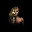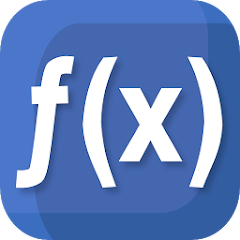# Mathematics

4.1
47.9K reviews
5M+Everyone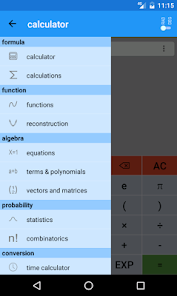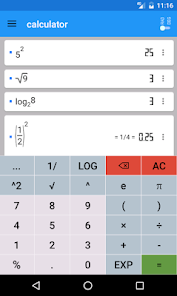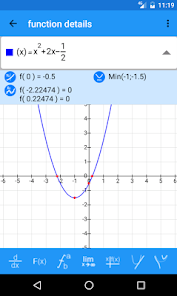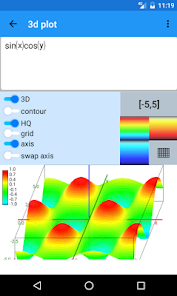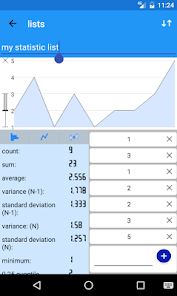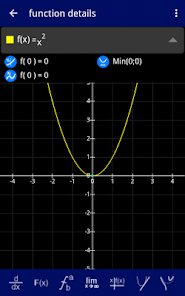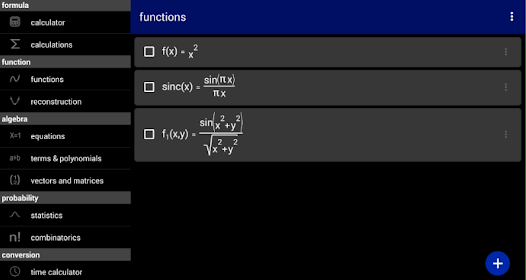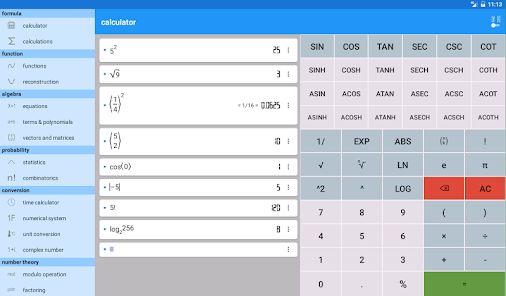Advanced Calculator for school or study that allows you to calculate formulas, solve equations or plot functions.

FEATURES

Calculate any formula you want and show them in a 2d or 3d plot. The natural display shows fractions, roots and exponents as you would expect it from mathematics.

In a few seconds you derivate or integrate your desired function, calculate the zero points of your function und show them in the function plot. See all maxima, minima or inflection points in one view.

The easy way of use allows you to solve linear equations in just a moment. Or transform your mathematical, physical or chemical equation to any unknown variable.

You often needs to calculate with binary, octal or hexadecimal number systems? No problem! You can mix them together in one calculation even by using decimal digits. But that's not enough! You can also calculate with any other number system with base 2 to 18.

From time to time you may need to convert units to another one, like Celsius to Fahrenheit, miles to kilometre, inches to foot and so on.

You will also be able to calculate with vectors, matrices and determinants.

All this features are combined in this app and will make your mathematical life a lot easier.
Updated on
Oct 7, 2019

## Data safetyNo information available

## Ratings and reviews

4.1
45.5K reviews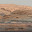Thaumaturge
November 16, 2020
Wonderful calculator! Need a simple calculator with more power than the basic app on your phone? This is it. Need a calculator app that doesn't have ads, very minimal memory footprint, and doesn't seem to track your every move? This is it. Minor learning curve for learning controls for selecting and managing inputs, but quickly overcome with generous use of parentheses.
659 people found this review helpful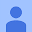August 25, 2019
This app is great. Besides doing simple calculations, it can also do complex calculations such as solving equations, plotting graphs, matrices and many other. It also shows expressions as real mathematical expressions. The only problem is that, the 3D graphs cannot be rotated and scaled. Please add that feature.
832 people found this review helpful